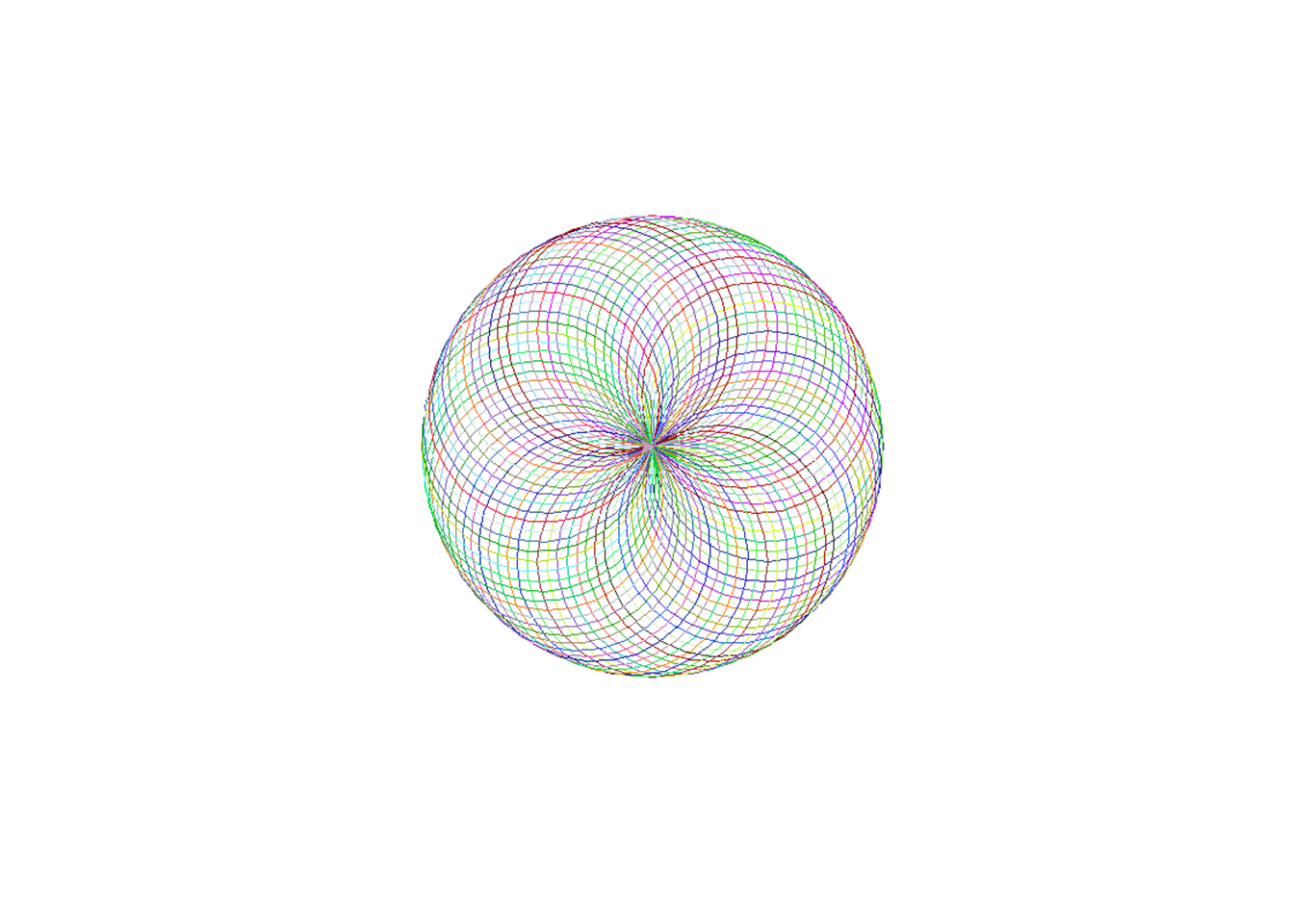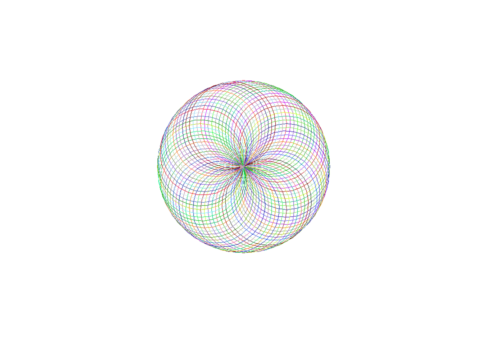# Spirograph in python with source code## Introduction

A Spirograph is a geometric drawing device that is used to draw a pattern of cyclical curved lines.  We can create our own Spirograph device using python turtles.

A Spirograph is a very interesting geometrical figure that has different colors. They are a bunch of circles overlapping each other and forming in a circular structure. In this tutorial, we are creating a spriograph drawing using python turtle graphics. Turtle graphics is a fun way of learning programming and using graphics in python programming. For creating a spriograph, we must import turtle, random library etc. See the code below.

```import turtle as t
import random

tim = t.Turtle()
t.colormode(255)

def random_color():
r = random.randint(0, 255)
g = random.randint(0, 255)
b = random.randint(0, 255)
color = (r, g, b)
return color

tim.speed("fastest")

def draw_spiograph(size_of_gap):
for _ in range(int(360 / size_of_gap)):
tim.color(random_color())
tim.circle(100)

draw_spiograph(5)

screen = t.Screen()
screen.exitonclick()```

output:

### How to use this Spirograph project?

• Copy the code and paste in your editor.
• Execute the code.
• See the output making.
• Enjoy and share.